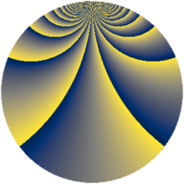# Properties

 Label 2736.2.cgLevel $2736$ Weight $2$ Character orbit 2736.cg Rep. character $\chi_{2736}(1151,\cdot)$ Character field $\Q(\zeta_{6})$ Dimension $80$ Newform subspaces $3$ Sturm bound $960$ Trace bound $19$

# Related objects

## Defining parameters

 Level: $$N$$ $$=$$ $$2736 = 2^{4} \cdot 3^{2} \cdot 19$$ Weight: $$k$$ $$=$$ $$2$$ Character orbit: $$[\chi]$$ $$=$$ 2736.cg (of order $$6$$ and degree $$2$$) Character conductor: $$\operatorname{cond}(\chi)$$ $$=$$ $$228$$ Character field: $$\Q(\zeta_{6})$$ Newform subspaces: $$3$$ Sturm bound: $$960$$ Trace bound: $$19$$

## Dimensions

The following table gives the dimensions of various subspaces of $$M_{2}(2736, [\chi])$$.

Total New Old
Modular forms 1008 80 928
Cusp forms 912 80 832
Eisenstein series 96 0 96

## Trace form

 $$80q + O(q^{10})$$ $$80q + 8q^{13} + 40q^{25} + 16q^{37} - 64q^{49} - 8q^{61} + 8q^{73} + 48q^{85} - 16q^{97} + O(q^{100})$$

## Decomposition of $$S_{2}^{\mathrm{new}}(2736, [\chi])$$ into newform subspaces

Label Dim. $$A$$ Field CM Traces $q$-expansion
$$a_2$$ $$a_3$$ $$a_5$$ $$a_7$$
2736.2.cg.a $$24$$ $$21.847$$ None $$0$$ $$0$$ $$0$$ $$0$$
2736.2.cg.b $$24$$ $$21.847$$ None $$0$$ $$0$$ $$0$$ $$0$$
2736.2.cg.c $$32$$ $$21.847$$ None $$0$$ $$0$$ $$0$$ $$0$$

## Decomposition of $$S_{2}^{\mathrm{old}}(2736, [\chi])$$ into lower level spaces

$$S_{2}^{\mathrm{old}}(2736, [\chi]) \cong$$ $$S_{2}^{\mathrm{new}}(228, [\chi])$$$$^{\oplus 6}$$$$\oplus$$$$S_{2}^{\mathrm{new}}(684, [\chi])$$$$^{\oplus 3}$$$$\oplus$$$$S_{2}^{\mathrm{new}}(912, [\chi])$$$$^{\oplus 2}$$